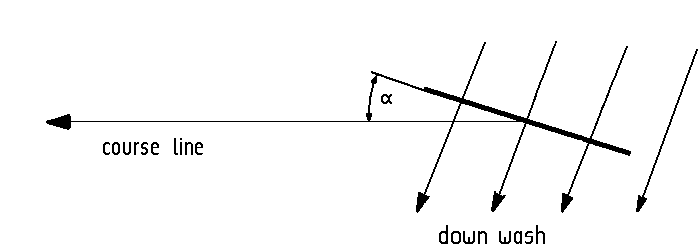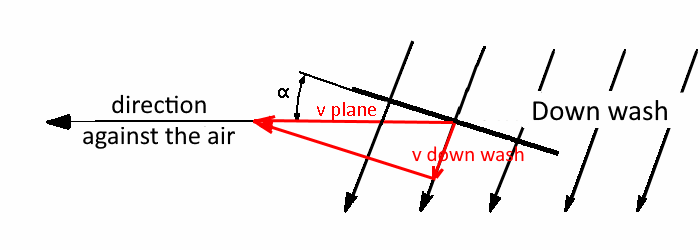Jan Peter Apel

The Basic Formula of Flying

The physical formula for lift differs in that, that lift does not come from an aerodynamic, but from an aerokinetic process. A wing draws air with its top towards it and presses air with its bottom down, both according to the principle of the inclined plane. The air sucked in from above combines behind the wing with the air pushed down from the bottom into a uniform air flow, the down wash, as the following sketch shows.The recoil force resulting from the acceleration of the air mass is calculated by Newton's force formula in the version with constant speed. It gives the force, that the air exerts against the wings.

F = m dt · v

The velocity of the down wash is:vdownwash   =   vplane  *  sin a

The vertical sink speed of the down wash, that is that speed of the air, that creates the lift force, is:vsink   =   vplane  * sin a  *  cos a

Now must determinate, how much the mass flow per second of the down wash is. It results from the density of the air times that area, that
the wings cover in one second times the vertical downward speed of the down wash. That is:

m dt  = rho * (vplane * 1 [s] * wing span) * ( vplane  *  sin a  *  cos a)

This mass of air flows vertically down through the lavel of the flight path. The reaction force from this mass is then calculated according to Newton's force formula by multiplying with the speed of the downward flowing air mass:

Recoil force = lift force =

density         flight area per second          velocity of down wash                  velocity of down wash
for the air mass                         for the recoil force
rho * (v plane * wing span) * (vplane * sin a * cos a) * (vplane * sin a * cos a) * 1[s]

Summarized the lift force L is:

L  =  rho · wing span · vplane3 · (sin α)2 · (cos α)2 · 1 [s]

This physically formula of flying is based on the air mass accelerated downwards by wings. How technology creates this with appropriate wing shapes and wing profiles is up to it. The angle of attack of the wing surfaces is decisive in any case. It alone is the basis of flying. What is happening in the air in the immediate vicinity of the wing profiles are only symptoms of what ultimately has to be done in order to be able to fly: behind all flying objects, especially the bumblebee too, air mass must flow down, only that has the corresponding repercussions for the development of the buoyancy force

That the airspeed is included with the third power is not an error. In free air space, however, an aircraft cannot achieve this state, since by increasing the angle of attack the increasing lift deflects the trajectory upward, so that the angle of attack drops again. In school, however, at last flying can be treated.